# Is the following vector field conservative? A(T (y 4x3 z, 2z3y s, 3y2z2 sin x -...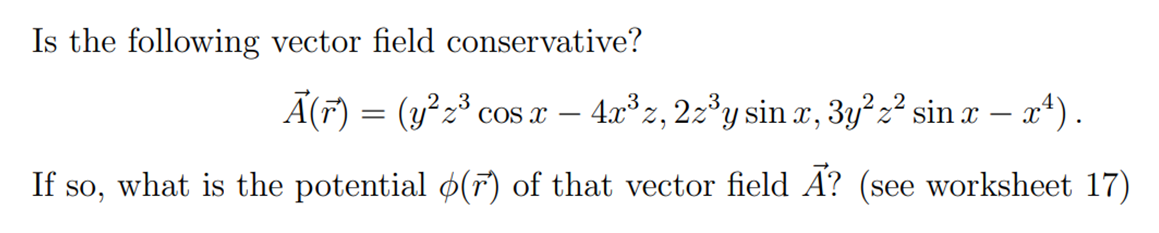Is the following vector field conservative? A(T (y 4x3 z, 2z3y s, 3y2z2 sin x - a) COS x _ If so, what is the potential ø(F) of that vector field A? (see worksheet 17)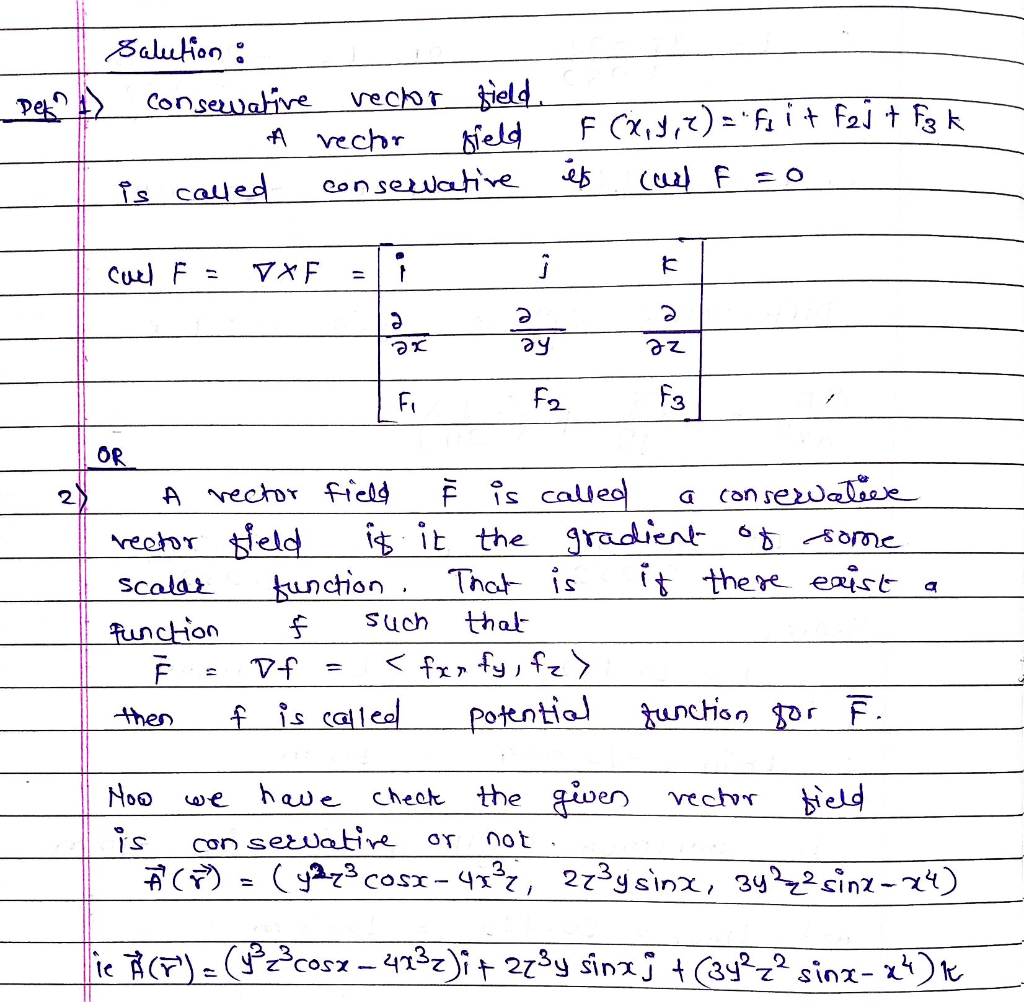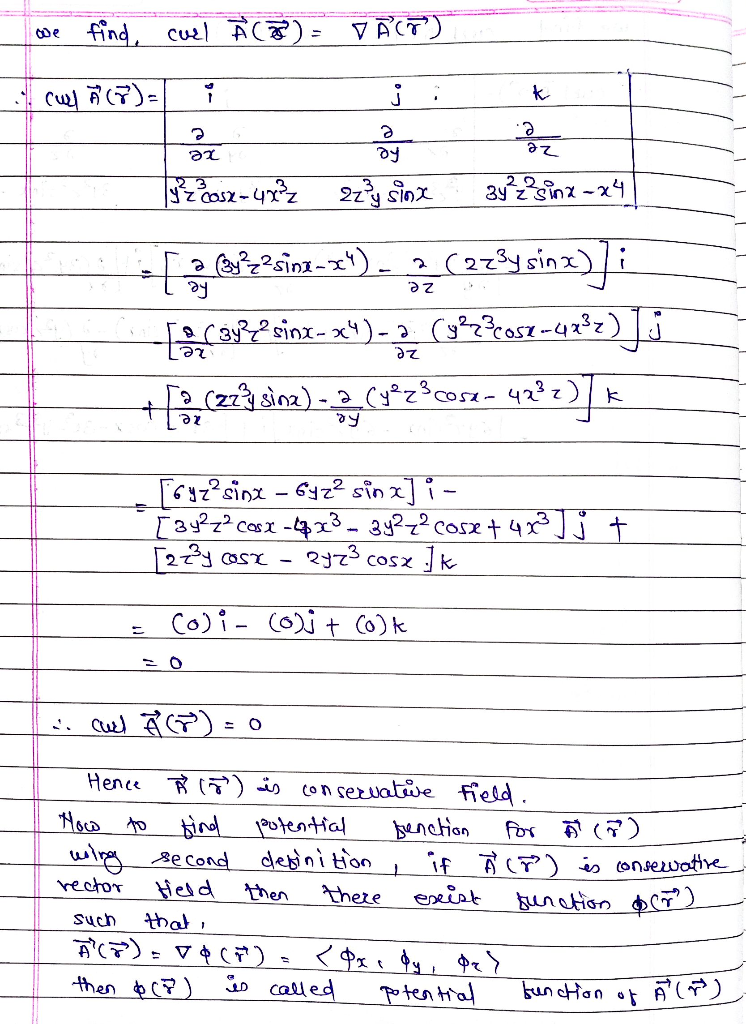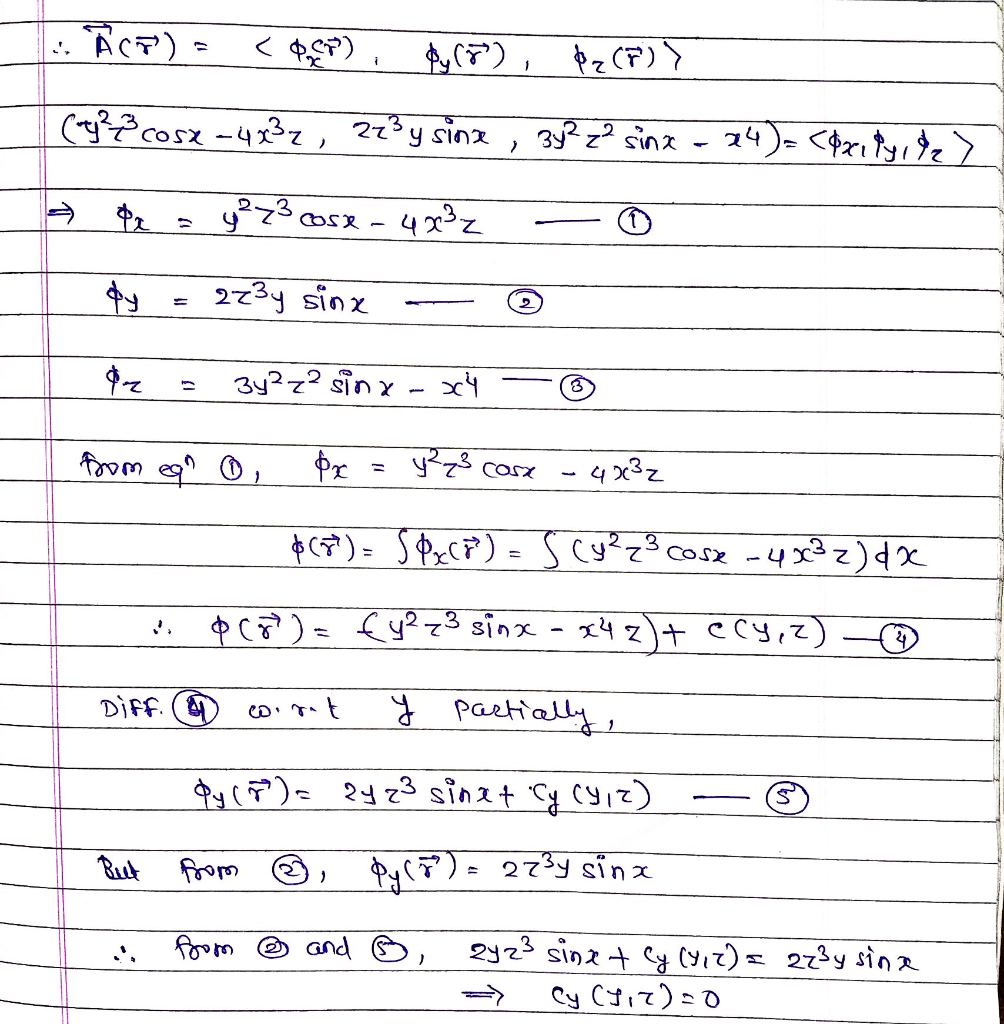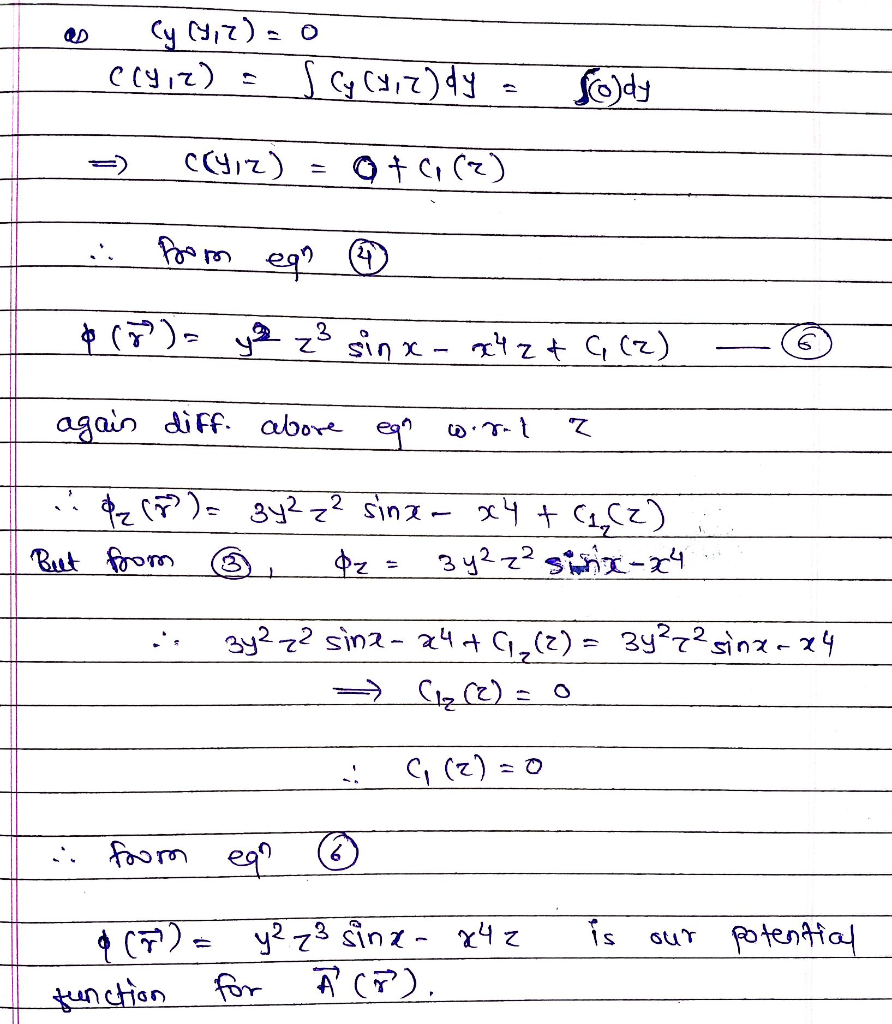#### Earn Coin

Coins can be redeemed for fabulous gifts.

Similar Homework Help Questions
• ### (1 point) Determine whether the vector field is conservative and, if so, find the general potential...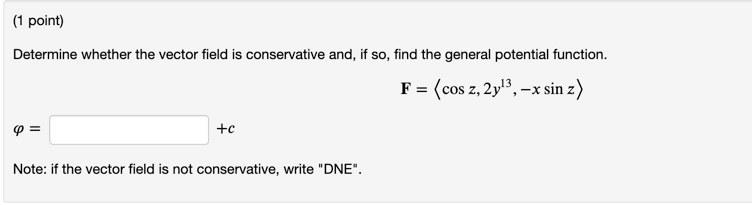(1 point) Determine whether the vector field is conservative and, if so, find the general potential function. F = (cos z, 2y!}, -x sin z) Q= +c Note: if the vector field is not conservative, write "DNE". (1 point) Show F(x, y) = (8xy + 4)i + (12x+y2 + 2e2y)j is conservative by finding a potential function f for F, and use f to compute SF F. dr, where is the curve given by r(t) = (2 sinº 1)i +...

• ### Let F(x, y, z) = sin yi + (x cos y + cos z)j – ysin...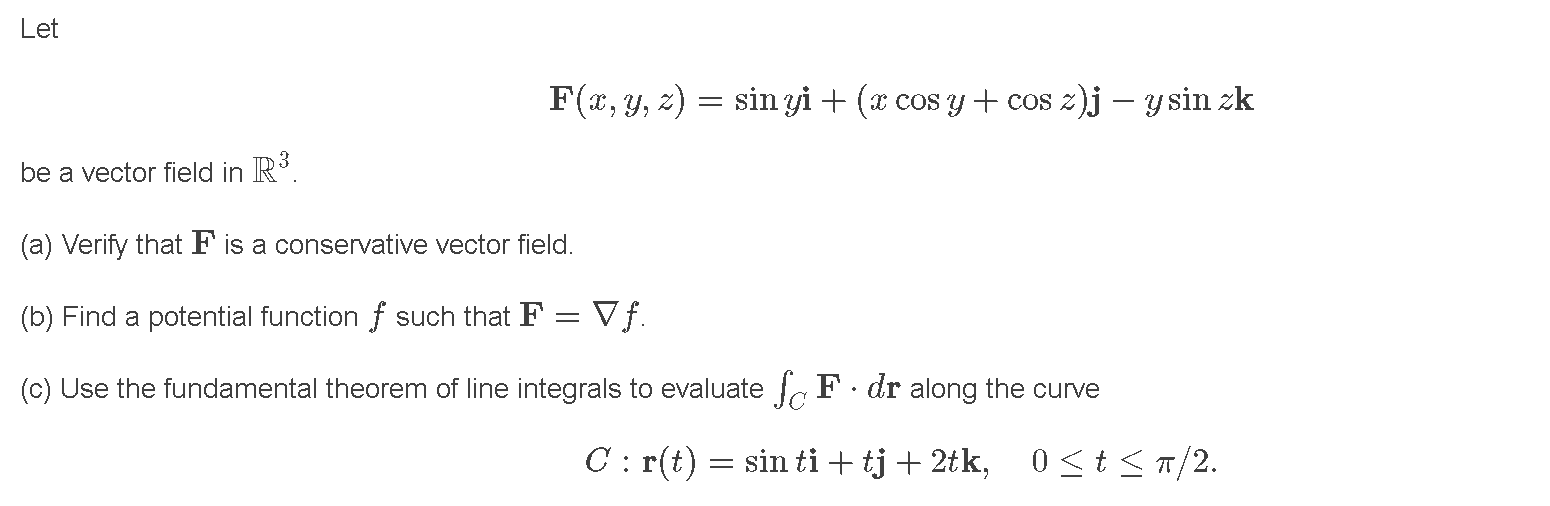Let F(x, y, z) = sin yi + (x cos y + cos z)j – ysin zk be a vector field in R3. (a) Verify that F is a conservative vector field. (b) Find a potential function f such that F = Vf. (C) Use the fundamental theorem of line integrals to evaluate ScF. dr along the curve C: r(t) = sin ti + tj + 2tk, 0 < t < A/2.

• ### 3. 8p] Show that the force field F(x,y, z) sin y, x cos y + cos z, -y sin z) is conservative and use this fact to evalu...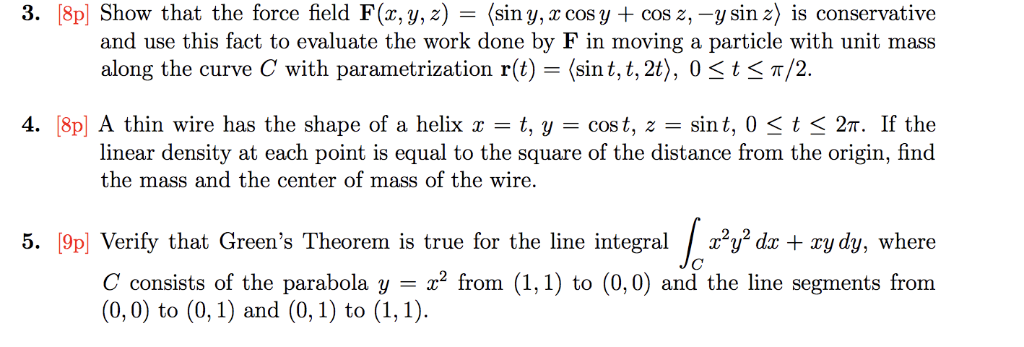3. 8p] Show that the force field F(x,y, z) sin y, x cos y + cos z, -y sin z) is conservative and use this fact to evaluate the work done by F in moving a particle with unit mass along the curve C with parametrization r(t (sin t, t, 2t), 0 <t<T/2. 4. 8p] A thin wire has the shape of a helix x = sin t, 0 < t < 27r. If the t, y = cos t,...

• ### 8Two vector fields are given: F(x,y,z) - (esin(yz), ze* cos(yz), ye* cos(yz)) and F(x,y,z) = (z...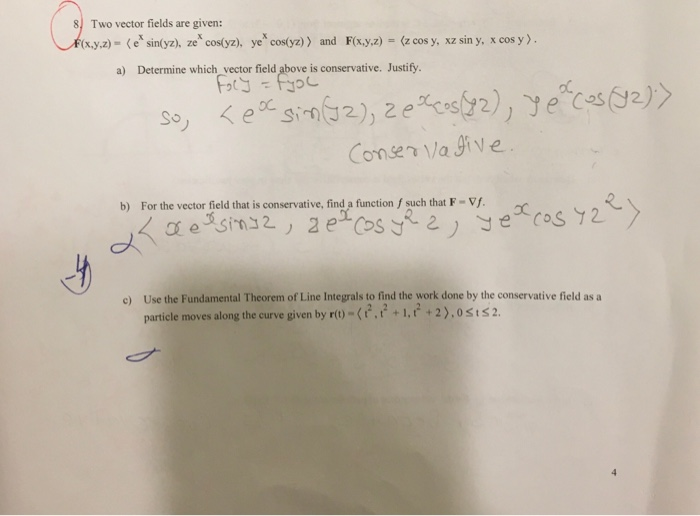8Two vector fields are given: F(x,y,z) - (esin(yz), ze* cos(yz), ye* cos(yz)) and F(x,y,z) = (z cos y, xz sin y, x cos y). a) Determine which vector field above is conservative. Justify. Foly = fjol so, <ea sin(J2), 20% cos(82), y acos (92)) Conservative. b) For the vector field that is conservative, find a function f such that F - Vf. Lxelsing2, zetos yea, yet cosy 2 c) Use the Fundamental Theorem of Line Integrals to find the work...

• ### Decide whether or not the vector field is a gradient field (i.e. is conservative). If it is conservative, find a potential function. (ii) F(x,y)-6ญ่-12xVJ (iv) F(x, y, z)-< ye", e + z,y >...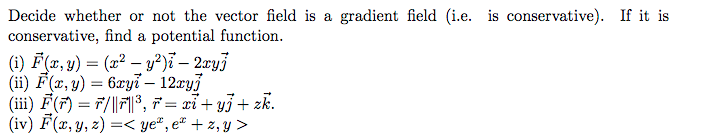Decide whether or not the vector field is a gradient field (i.e. is conservative). If it is conservative, find a potential function. (ii) F(x,y)-6ญ่-12xVJ (iv) F(x, y, z)-< ye", e + z,y > Decide whether or not the vector field is a gradient field (i.e. is conservative). If it is conservative, find a potential function. (ii) F(x,y)-6ญ่-12xVJ (iv) F(x, y, z)-

• ### Consider the vector field. F(x, y, z) = (3ex sin(y), 3ey sin(z), 5e7 sin(x)) (a) Find...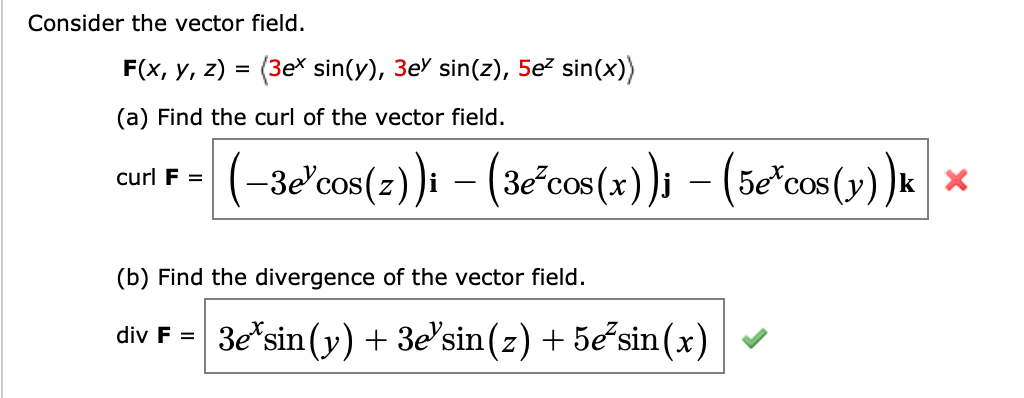Consider the vector field. F(x, y, z) = (3ex sin(y), 3ey sin(z), 5e7 sin(x)) (a) Find the curl of the vector field. curl F = (-3d"cos(z))i – (36*cos(x)); – (5e+cos(y) )* * (b) Find the divergence of the vector field. div F = 3e'sin(y) + 3e'sin(z) + 5e+ sin(x)

• ### Question 2 For the following vector fields F determine whether or not they are conservative. For ...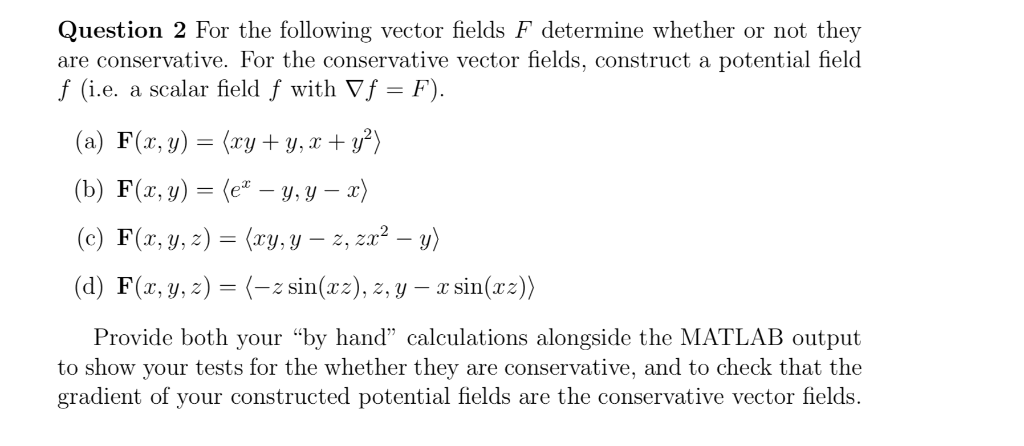Only the Matlab part !!! Question 2 For the following vector fields F determine whether or not they are conservative. For the conservative vector fields, construct a potential field f (i.e. a scalar field f with Vf - F) (a) F(z, y)(ryy,) (b) F(z, y)-(e-y, y-z) (c) F(r, y,z) (ry.y -2, 22-) (d) F(x, y, z)=(-, sin(zz),2, y-rsin(x:) Provide both your "by hand" calculations alongside the MATLAB output to show your tests for the whether they are conservative, and to...

• ### + cos(y) is conservative by responding to the 2. Show that the vector field F(x,y) =...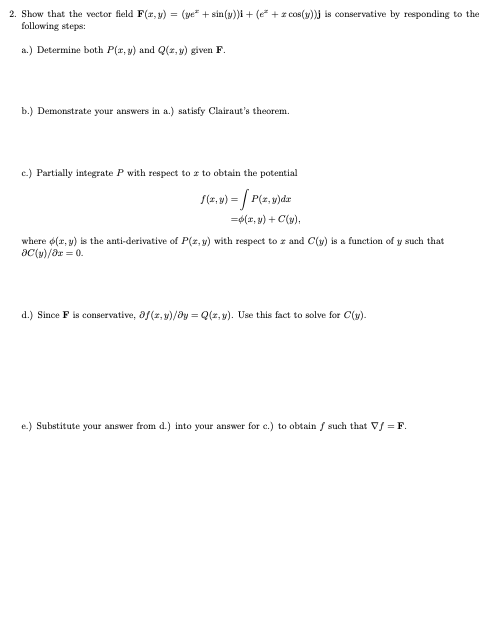+ cos(y) is conservative by responding to the 2. Show that the vector field F(x,y) = (ye* + sin(y))i + ( following steps: a.) Determine both P(x,y) and Q(x,y) given F. b.) Demonstrate your answers in a.) satisfy Clairaut's theorem. c.) Partially integrate P with respect to r to obtain the potential S(= y) = P(x,y)da = (1.x) + C) where (a,b) is the anti-derivative of P(x,y) with respect to r and C(y) is a function of y such that...

• ### = Consider the vector field F(x, y) (cos y + y cos x)i + (sin x...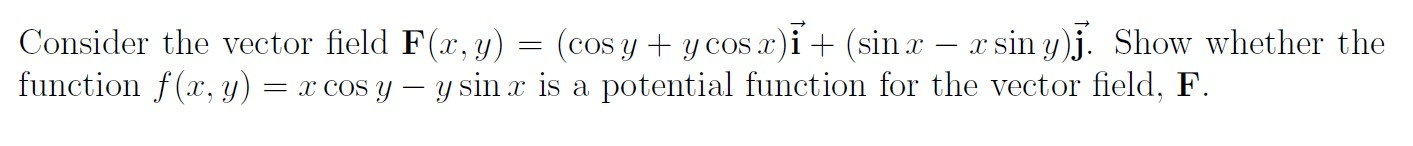= Consider the vector field F(x, y) (cos y + y cos x)i + (sin x – xsin y)j. Show whether the function f(x,y) = x COS Y – y sin x is a potential function for the vector field, F.

• ### Evaluate the line integral f F dr for the vector field F(x, y, z) curve C...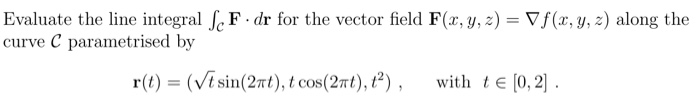Evaluate the line integral f F dr for the vector field F(x, y, z) curve C parametrised by Vf (x, y, z) along the with tE [0, 2 r() -(Vt sin(2πt), t cos (2πi), ?) , Evaluate the line integral f F dr for the vector field F(x, y, z) curve C parametrised by Vf (x, y, z) along the with tE [0, 2 r() -(Vt sin(2πt), t cos (2πi), ?) ,

Free Homework App